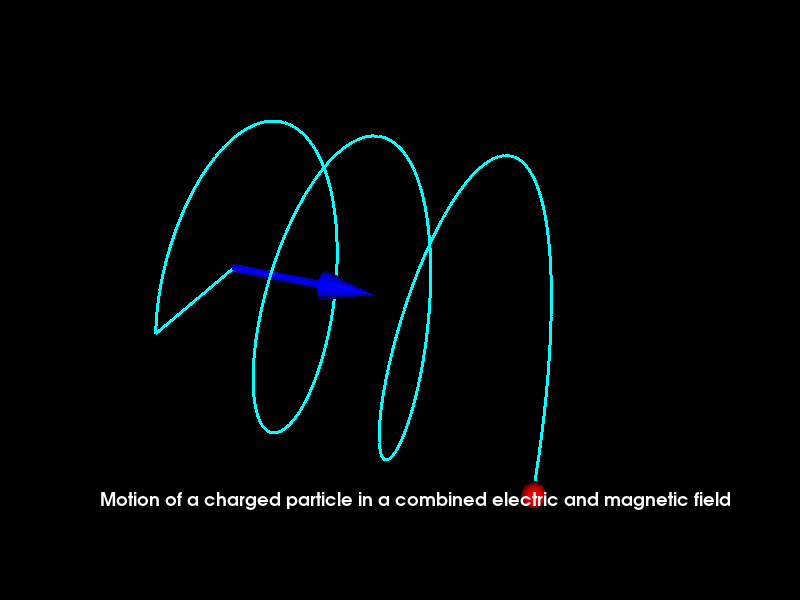# Motion of a charged particle in a combined magnetic and electric field#

A charged particle follows a curved path in a magnetic field. In an electric field, the particle tends to accelerate in a direction parallel/antiparallel to the electric field depending on the nature of charge on the particle. In a combined electric and magnetic field, the particle moves along a helical path.

In this animation, there’s a magnetic and an electric field present in +x direction under whose influence the positively charged particle follows a helical path.

Importing necessary modules

```import itertools

import numpy as np

from fury import actor, ui, utils, window
```

Let’s define some variable and their description:

• radius_particle: radius of the point that will represent the particle (default = 0.08)

• initial_velocity: initial velocity of the particle along +x (default = 0.09)

• acc: acceleration of the particle along +x (due to the electric field) (default = 0.004)

• time: time (default time i.e. time at beginning of the animation = 0)

• incre_time: value by which time is incremented for each call of timer_callback (default = 0.09)

• angular_frq: angular frequency (default = 0.1)

• phase_angle: phase angle (default = 0.002)

```radius_particle = 0.08
initial_velocity = 0.09
acc = 0.004
time = 0
incre_time = 0.09
angular_frq = 0.1
phase_angle = 0.002
```

Creating a scene object and configuring the camera’s position

```scene = window.Scene()
scene.zoom(1.2)
scene.set_camera(
position=(10, 12.5, 19), focal_point=(3.0, 0.0, 0.0), view_up=(0.0, 0.0, 0.0)
)
showm = window.ShowManager(
scene, size=(800, 600), reset_camera=True, order_transparent=True
)
```

Creating a blue colored arrow which shows the direction of magnetic field and electric field.

```color_arrow = window.colors.blue  # color of the arrow can be manipulated
centers = np.array([[0, 0, 0]])
directions = np.array([[1, 0, 0]])
heights = np.array()
arrow_actor = actor.arrow(
centers,
directions,
color_arrow,
heights,
resolution=20,
tip_length=0.06,
)
```

Initializing the initial coordinates of the particle

```x = initial_velocity * time + 0.5 * acc * (time**2)
y = np.sin(angular_frq * time + phase_angle)
z = np.cos(angular_frq * time + phase_angle)
```

Initializing point actor which will represent the charged particle

```color_particle = window.colors.red  # color of particle can be manipulated
pts = np.array([[x, y, z]])

vertices = utils.vertices_from_actor(charge_actor)
vcolors = utils.colors_from_actor(charge_actor, 'colors')
no_vertices_per_point = len(vertices)
initial_vertices = vertices.copy() - np.repeat(pts, no_vertices_per_point, axis=0)
```

Initializing text box to display the name of the animation

```tb = ui.TextBlock2D(bold=True, position=(100, 90))
m1 = 'Motion of a charged particle in a '
m2 = 'combined electric and magnetic field'
tb.message = m1 + m2
```

Initializing counter

```counter = itertools.count()
```

end is used to decide when to end the animation

```end = 200
```

This will be useful for plotting path of the particle

```coor_1 = np.array([0, 0, 0])
```

Coordinates to be plotted are changed every time timer_callback is called by using the update_coordinates function. The wave is rendered here.

```def timer_callback(_obj, _event):
global pts, time, incre_time, coor_1
time += incre_time
cnt = next(counter)

x = initial_velocity * time + 0.5 * acc * (time**2)
y = np.sin(10 * angular_frq * time + phase_angle)
z = np.cos(10 * angular_frq * time + phase_angle)
pts = np.array([[x, y, z]])

vertices[:] = initial_vertices + np.repeat(pts, no_vertices_per_point, axis=0)

utils.update_actor(charge_actor)

# Plotting the path followed by the particle
coor_2 = np.array([x, y, z])
coors = np.array([coor_1, coor_2])
coors = [coors]
line_actor = actor.line(coors, window.colors.cyan, linewidth=3)
coor_1 = coor_2

showm.render()

# to end the animation
if cnt == end:
showm.exit()
```

Run every 15 milliseconds

```showm.add_timer_callback(True, 15, timer_callback)
showm.start()
window.record(showm.scene, size=(800, 600), out_path='viz_helical_motion.png')
```Total running time of the script: ( 0 minutes 5.003 seconds)

Gallery generated by Sphinx-Gallery